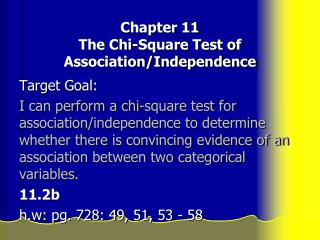DownloadDownload PresentationChapter 11 The Chi-Square Test of Association/Independence

# Chapter 11 The Chi-Square Test of Association/Independence

Download Presentation## Chapter 11 The Chi-Square Test of Association/Independence

- - - - - - - - - - - - - - - - - - - - - - - - - - - E N D - - - - - - - - - - - - - - - - - - - - - - - - - - -
##### Presentation Transcript

1. Chapter 11The Chi-Square Test of Association/Independence Target Goal: I can perform a chi-square test for association/independence to determine whether there is convincing evidence of an association between two categorical variables. 11.2b h.w: pg. 728: 49, 51, 53 - 58

2. The chi-square test can also be used to show evidence that there is a relationship between two categorical variables. • Use this if you have independent SRS’s from several populations where one variable is categorical and the other is the sample number. • Or, if you have a single SRS with each individual classified according to two categorical variables. • Or, if you have an entire population with each individual classified according to two categorical variables.

3. Ex: Smoking and SES An example that classifies observations from a single population in two ways: by smoking habits and SES. • In a study of heart disease in male federal employees, researchers classified 356 volunteer subjects according to their socioeconomic status (SES) and their smoking status.

4. Observed Counts for smoking and SES SES Smoking High Middle Low Total Current 51 22 43 116 Former 92 21 28 141 Never 68 9 22 99 Total 211 52 93 356 • This is a 3x3 table with added margin totals. Even though this example is different than comparing several proportions, we can still apply the chi-square test because the row and column variables are not related to each other.

5. The Chi-Square Test of Association/Independence Use the chi-square test of association/independence to test the null hypothesis, Ho: there is no relationship between two categorical variables when you have a two way table from a single SRS, with each individual is classified according to both oftwo categorical variables.

6. SES cont. • SES is the explanatory variable therefore we need to compare the column percents that give the conditional distribution of smoking within each SES category.

7. Calculate Column Percents: • 51/211 = 0.242 about 24.2% of the high-SES group are current smokers. • Fill in the rest of the table.

8. Column percents for Smoking and SES SES Smoking High Middle Low Current 24.2 42.3 46.2 Former 43.6 40.4 30.1 Never 32.2 17.3 23.7 Total 100.0 100.0 100.0 What do the column percents suggest?

9. There is a negative association between smoking and SES. • The lower the SES, the more likely to smoke.

10. Computing Expected Cell Counts • 116 x 211 = 68.75 356

11. Expected Count for Smoking and SES SES Smoking High Middle Low Total Current 68.75 16.94 30.30 115.99 Former 83.57 20.60 36.83 141.00 Never 58.68 14.46 25.86 99.00 Total 211 52 92.99 355.99

12. Chi-square Test for Association/Independence Step 1: State - We want to perform a test of Ho: There is no association between smoking and SES. Ha: There is an association between smoking and SES.

13. Step 2: Plan If conditions are met, we should carry out a chi-square test of association/independence. Random: The subjects were volunteers, we may not be able to generalize our results. Large Sample Size: • To use chi-square we must check all expected counts. • We did this and all counts ≥ 1 and no more than 20% < 5.

14. Independence: • Because we are sampling without replacement, we need to check the 10% condition.It is safe to assume that the total number of male federal employees is at least 10(356) = 3560. • Thus, knowing the values of both variables for one person gives us no meaningful information about the variables for another person. So, individual observations are independent.

15. Step 3: Carry out the inference procedure. • The test statistic • Calculate by hand with df = (r-1)(c-1) = • Or with calculator, need to enter observed counts into matrix table A. • Note: the calculator will calculate the expected counts for you when you execute the X2 test.

16. Note: if doing by hand, could write calculator program to do “expected counts” or must do by hand. • Enter observed values in matrix A, • Then STAT:TESTS: -Test • The calculator enters expected values in matrix B. • P-value = .00098 Note: the association does not mean that SES causes smoking behavior.

17. Step 4: Conclude –Interpret the results in context. • With a p-value this low, we reject the null hypothesis at the alpha = .01 level and conclude that there is strong evidence of an association between smoking and SES in the population of male federal employees.

18. Computer Output

19. Follow-up Analysis Inference for Relationships Start by examining which cells in the two-way table show large deviations between the observed and expected counts. Then look at the individual components to see which terms contribute most to the chi-square statistic. Minitab output for the wine and music study displays the individual components that contribute to the chi-square statistic.

20. Follow-up Analysis Inference for Relationships Looking at the output, we see that just two of the nine components that make up the chi-square statistic contribute about 14 (almost 77%) of the total χ2 = 18.28. We are led to a specific conclusion: sales of Italian wine are strongly affected by Italian and French music.